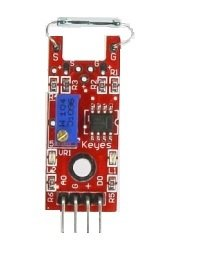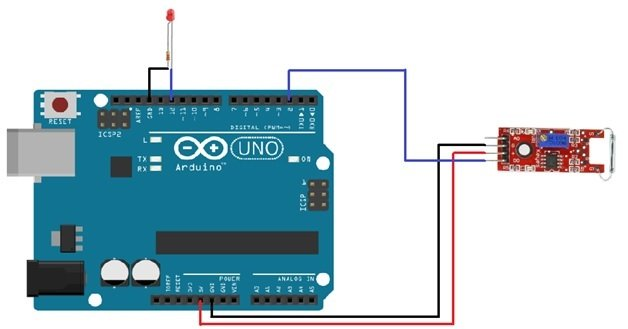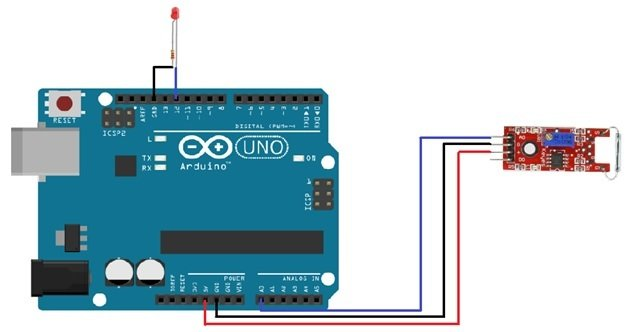# reed switch interfacing with arduino|magnetic field dectection

Reed Switch Module :Reed switch is basically an electrical switch which is operated when a magnetic field is brought near to it. It is constructed by using two small metal plates within a glass tube under vacuum. When detecting the magnetic field the sheets come together and close the circuit. The switch will be activated when there will be magnetic field near it. Reed switch is basically used to detect the magnetic field.

In this tutorial we will use this switch to light up a led when a magnetic field will be detected. The basic difference between the reed switch module and mini reed switch module is that the reed switch module gives us both the analog and digital outputs while the mini reed switch gives us only digital output. We are going to discuss both.

### what is Reed Switch

This switch provides us an analog as well as a digital interface. You can see that the reed switch module has a potentiometer that is used as a pull up resistor. The module also has an on board led which indicates that the reed switch has detected a magnet field or not. These are just used for the switching mechanism using electromagnetism. The reed switch module has both analog and digital output. So, we can use it in both ways. In this tutorial, we are going to use it in both ways.

### reed switch Pin Out

The pin out of the reed switch is as followsA0: analog pin, this will be connected to the analog pin of Arduino.

G: ground, this will be connected to the ground of the Arduino.

+: 5V pin. This will be connected to 5V pin of the Arduino.

D0: Digital pin, this will be connected to any digital pin of the Arduino.

### reed switch interfacing with Arduino digital pinThe connections are very easier. We have connected the +5V and ground pin of the module with the +5v and the ground pin of the Arduino. Then we connected the digital of the module with the pin 2 of the Arduino. A led is attached at the pin 12 of the Arduino which will light up when the module will sense a magnetic field. While using the digital pin, we can change the threshold value by changing the resistance of potentiometer (rotating the top of potentiometer).

### Code of reed switch with arduino

// This code is for testing the  switch when using the digital pin

```int Led_pin = 12 ;                                              // initializing the pin 12 as led pin

int Sensor_pin = 2 ;                                         // initializing the pin 2 sensor pin

int Value ;                                        // initializing a variable to store  sensor output

void setup ( )

{

pinMode ( Led_pin, OUTPUT ) ;                               // declaring pin 12 as output pin

pinMode ( Sensor_pin, INPUT ) ;            // declaring pin 2 as input pin

}

void loop ( )

SunFounder {

Value = digitalRead ( Sensor_pin ) ;        // reading the sensor state

if (Value == HIGH )                                        // If sensor pin is high then high the pin 12

{

digitalWrite ( Led_pin, HIGH ) ;

}

else

{

digitalWrite ( Led_pin, LOW ) ;                // Otherwise turn off or LOW

}

}```

### switch interfacing with Arduino analog pinThe connections are same like we used for digital pin excluding the use of digital pin is replaced by the analog pin. The Arduino gives us output from 0 to 1023 and we will show these values on the serial monitor.

### Code of reed switch interfacing with arduino

// This code is for testing the  switch when using the analog pin

```int Sensor_pin = A0 ;                                                      // initializing A0 as sensor pin

int Led_pin = 12 ;                                                              // initializing pin 12 as led pin

int Value = 0 ;                                                                     // initializing a variable to store sensor value

void setup ( ) {

pinMode ( Led_pin, OUTPUT ) ;                                               // declaring pin 12 as output pin

Serial.begin ( 9600 ) ;                                                    // Setting baud rate at 9600

}

void loop ( ) {

Value = analogRead ( Sensor_pin ) ;                      // reading from the sensor

digitalWrite ( Led_pin, HIGH ) ;

delay (Value ) ;

digitalWrite ( Led_pin, LOW ) ;

delay ( Value ) ;

Serial.print( "SENSOR PIN A0:" )

Serial.println ( Value, DEC ) ;                                                      // displaying the value on serial monitor

}```

### Mini Reed Switch

Unlike the switch which has both analog and digital output, mini reed switch has only one output.

Pin Out

The pin out of the mini reed switch from left to right is as follows.

S: signal pin

+5V: 5 volt pin

Gnd: ground pin

### Code of mini reed switch interfacing with arduino

// This code is for testing the mini reed switch

```const int mini_reed_swtich_pin = 12;                                      // declaring pin 12 as the switch pin

const int led_pin = 9;                                                    // declaring pin 9 as the led pin

int store_value = 0;                                                         // variable to store the value

void  setup ( )  {

pinMode (led_pin, OUTPUT );                                                    // declaring led pin as output pin

pinMode (mini_reed_swtich_pin, INPUT );                          // declaring switch pin as input pin

}

void  loop () {

if (store_value == HIGH ) {                              // checks whether state of switch is high or not

digitalWrite (led_pin, HIGH );                             // turning on the led if the switch state is high

}

else {

digitalWrite (led_pin, LOW );                                                                       // Otherwise turning it low

}

}```

### 1 thought on “reed switch interfacing with arduino|magnetic field dectection”

1. Hi,
Im busy with a project where Im using Neopixel strip, Adafruit board and a mini Reed switch, and I need some help with the coding as I am still very new to this.
What I want to do is say for example:
if the switch is open, then LED 0 – 25 is white, if the switch is closed then LED 0 – 25 turns green.

What would the best way to code this be?